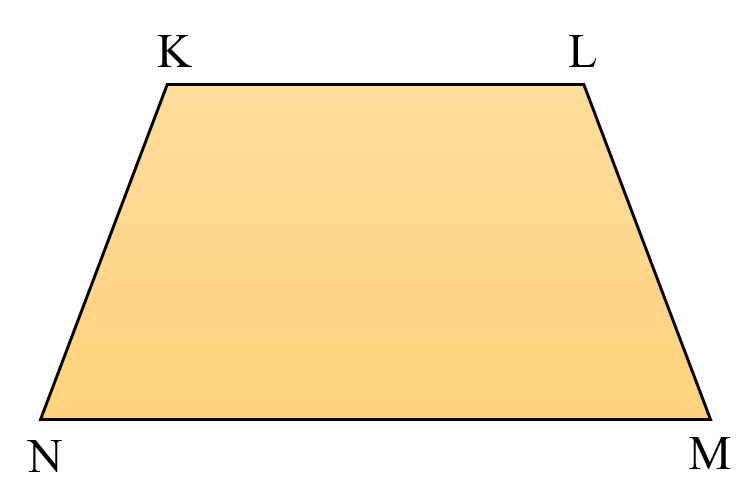# vs.eyeandcontacts.com

## Chapter 4 Basic Geometrical Ideas Exercise 4.5

Question 1: Draw a rough sketch of a quadrilateral PQRS. Draw its diagonals. Name them. Is the meeting point of the diagonals in the interior or exterior of the quadrilateral?PR and QS are the diagonals. These two diagonals meet at one point, O, which lies in the interior of the quadrilateral PQRS.

Question 2: Draw a rough sketch of a quadrilateral KLMN. State,
a) two pairs of opposite sides,
b) two pairs of opposite angles,
c) two pairs of adjacent sides,
d) two pairs of adjacent angles.a)
KL and NM
KN and LM

b)
∠KNM, ∠KLM
∠LKN, ∠LMN

c)
KN, KL
KN, NM
LK, LM
ML, MN

d)
∠K, ∠L
∠M, ∠N
∠K, ∠L
∠L, ∠M

Question 3: Investigate:
Use strips and fasteners to make a triangle and a quadrilateral. Try to push inward at any one vertex of the triangle. Do the same to the quadrilateral. Is the triangle distorted? Is the quadrilateral distorted? Is the triangle rigid? Why is it that structures like electric towers make use of triangular shapes and not quadrilaterals?